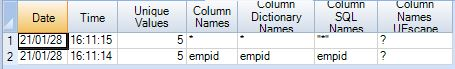Whenever the user executes any query, Teradata creates different execution plans and chooses the best plan to execute the SQL query. These execution plans are based on the statistics collected on the tables used within the SQL query.

The information collected by the `COLLECT STATISTIC` method stores in the Data Dictionary(DD) tables. This information is used by the Teradata optimizer during the creation of query execution plans and joins plans.

Followings are the information Teradata Collects using collect statistics methods.

Data Demographics

1. The number of rows in the table.
2. The average row size.
3. The range of values in the column(s) where statistics are collected.
4. Information about indexes in the table.
5. The number of rows per value for the column where statistics are collected.

Environment Information

1. The number of Nodes, AMPs, CPUs.
2. Amount of Memory.

The syntax to collect statistics as follows.

```COLLECT [SUMMARY] STATISTICS
INDEX(index_name)
COLUMN(column_name)
ON <table_name>;```

The following example collects statistics on the employee table.

`COLLECT STATISTICS COLUMN(empid) on Teradatapoint.employee;`

### Viewing Statistics

In Teradata you can check about the statistical information like on which column(s) and when statistics have been collected. You can view the statistics information using the HELP STATISTICS command.

Syntax

The following syntax shows the statistics collected on the table.

`HELP STATISTICS <table_name>;`

Example

In the following example, we check the statistics information in the employee table.

`HELP STATISTICS Teradatapoint.employee;`

The result of the above query is as below.Teradata stores all the collect statistics information in the DBC in the dictionary tables. Those are –

1. DBC.Indexes (for multicolumn index only)
2. DBC.TVFields (for all columns and single column indexes)
3. DBC.statsTbl (Teradata v14 and beyond)